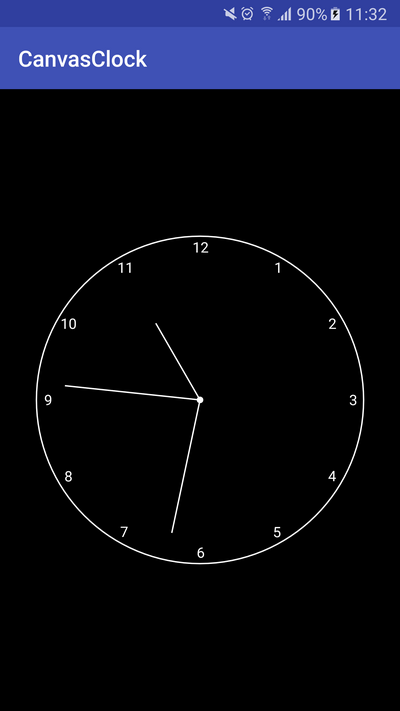To learn to use the Canvas 2D API, a good exercise is to draw an Analog Clock on Android. In this tutorial, you are going to discover how to create this kind of Clock and you will see some basics like creating a custom view and draw on a Canvas.

Note that you can enjoy this tutorial in video on Youtube :

The first step of the tutorial is to create a custom ClockView which will be used to draw the Analog Clock on the screen. To start, you need to define an initClock() method where you get the height and the width of the custom view. The minimum between the height and the width will be used to determine the dimensions of the square nesting the Analog Clock.

For the Analog Clock, we will define the radius by dividing the min between the height and the width by two. We subtract a padding value used to add some padding for the Analog Clock.

To draw the Analog Clock, we need to override the onDraw() method of our ClockView. If the view is not initialized, we call the initClock(). Then, we call the methods defined to draw the circle of the clock, the center, the numerals and the hands.

To update the time displayed on the Clock, we need to invalidate the view. Thus, it is drawn each 500 milliseconds.

It gives us the following code for the ClockView :

```
package com.ssaurel.canvasclock;

import android.content.Context;
import android.graphics.Canvas;
import android.graphics.Color;
import android.graphics.Paint;
import android.graphics.Rect;
import android.util.AttributeSet;
import android.util.TypedValue;
import android.view.View;

import java.util.Calendar;

public class ClockView extends View {

private int height, width = 0;
private int fontSize = 0;
private int numeralSpacing = 0;
private int handTruncation, hourHandTruncation = 0;
private Paint paint;
private boolean isInit;
private int[] numbers = {1,2,3,4,5,6,7,8,9,10,11,12};
private Rect rect = new Rect();

public ClockView(Context context) {
super(context);
}

public ClockView(Context context, AttributeSet attrs) {
super(context, attrs);
}

public ClockView(Context context, AttributeSet attrs, int defStyleAttr) {
super(context, attrs, defStyleAttr);
}

private void initClock() {
height = getHeight();
width = getWidth();
fontSize = (int) TypedValue.applyDimension(TypedValue.COMPLEX_UNIT_SP, 13,
getResources().getDisplayMetrics());
int min = Math.min(height, width);
handTruncation = min / 20;
hourHandTruncation = min / 7;
paint = new Paint();
isInit = true;
}

@Override
protected void onDraw(Canvas canvas) {
if (!isInit) {
initClock();
}

canvas.drawColor(Color.BLACK);
drawCircle(canvas);
drawCenter(canvas);
drawNumeral(canvas);
drawHands(canvas);

postInvalidateDelayed(500);
invalidate();
}

private void drawHand(Canvas canvas, double loc, boolean isHour) {
double angle = Math.PI * loc / 30 - Math.PI / 2;
canvas.drawLine(width / 2, height / 2,
(float) (width / 2 + Math.cos(angle) * handRadius),
(float) (height / 2 + Math.sin(angle) * handRadius),
paint);
}

private void drawHands(Canvas canvas) {
Calendar c = Calendar.getInstance();
float hour = c.get(Calendar.HOUR_OF_DAY);
hour = hour > 12 ? hour - 12 : hour;
drawHand(canvas, (hour + c.get(Calendar.MINUTE) / 60) * 5f, true);
drawHand(canvas, c.get(Calendar.MINUTE), false);
drawHand(canvas, c.get(Calendar.SECOND), false);
}

private void drawNumeral(Canvas canvas) {
paint.setTextSize(fontSize);

for (int number : numbers) {
String tmp = String.valueOf(number);
paint.getTextBounds(tmp, 0, tmp.length(), rect);
double angle = Math.PI / 6 * (number - 3);
int x = (int) (width / 2 + Math.cos(angle) * radius - rect.width() / 2);
int y = (int) (height / 2 + Math.sin(angle) * radius + rect.height() / 2);
canvas.drawText(tmp, x, y, paint);
}
}

private void drawCenter(Canvas canvas) {
paint.setStyle(Paint.Style.FILL);
canvas.drawCircle(width / 2, height / 2, 12, paint);
}

private void drawCircle(Canvas canvas) {
paint.reset();
paint.setColor(getResources().getColor(android.R.color.white));
paint.setStrokeWidth(5);
paint.setStyle(Paint.Style.STROKE);
paint.setAntiAlias(true);
canvas.drawCircle(width / 2, height / 2, radius + padding - 10, paint);
}

}

```

Once the ClockView is created, we have just to add it in the layout of our main activity :

```
<?xml version="1.0" encoding="utf-8"?>
<RelativeLayout xmlns:android="http://schemas.android.com/apk/res/android"
xmlns:tools="http://schemas.android.com/tools"
android:id="@+id/activity_main"
android:layout_width="match_parent"
android:layout_height="match_parent"
tools:context="com.ssaurel.canvasclock.MainActivity"
android:background="@android:color/black"
>

<com.ssaurel.canvasclock.ClockView
android:layout_width="300dp"
android:layout_height="300dp"
android:layout_centerInParent="true"/>

</RelativeLayout>

```

The code of the Main Activity is simple. We have just to set this layout as the content view :

```
package com.ssaurel.canvasclock;

import android.support.v7.app.AppCompatActivity;
import android.os.Bundle;

public class MainActivity extends AppCompatActivity {

@Override
protected void onCreate(Bundle savedInstanceState) {
super.onCreate(savedInstanceState);
setContentView(R.layout.activity_main);
}
}

```

Now, you can try your Analog Clock application and you should enjoy the following result :